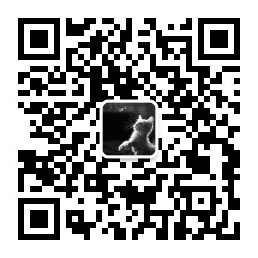# 【GNN】NGCF：捕捉协同信号的 GNN

• 时间:

Embedding 向量是现代推荐系统的核心，但是目前的方法无法捕捉到 user-item 交互中潜在的协作信号。因此，由此产生的 Embedding 向量可能不足以捕获到协同过滤的内容。

# 1.Introduction

1. Embedding：将 user 和 item 转换成向量表示。比如说矩阵分解得到 Embedding，并建模了 user 和 item 之间的交互信息；
2. 交互建模：基于 Embedding 重建历史交互。比如说利用非线性神经网络代替 MF 中的内积或者利用欧几里得作为交互函数；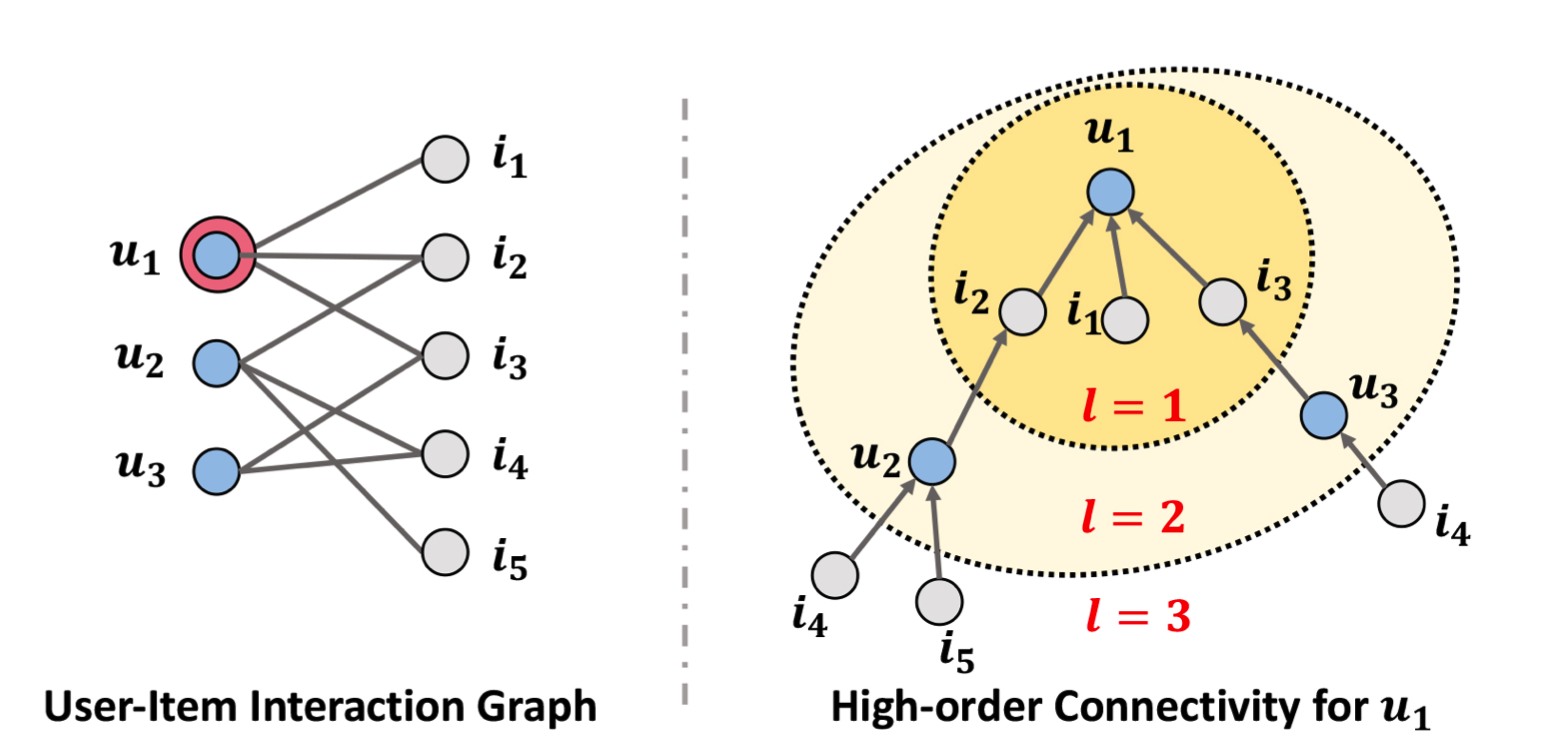# 2.NGCF

NGCF 总共有三个部分：Embedding Layer、Embedding Propagation Layers 和 Prediction Layer。

## 2.1 Embedding Layer

Embedding Layer 提供初始化的 user Embedding 和 item Embedding，可以被认为是构建了一个参数矩阵作为 Embedding look-up 表：
$\mathbf{E}=[\underbrace{\mathbf{e}_{u_1},…,\mathbf{e}_{u_N}}_{\text{users embedding}} , \underbrace{\mathbf{e}_{i_1},…,\mathbf{e}_{i_M}}_{\text{item embedding}}] \\$

## 2.2 Embedding Propagation Layers

### 2.2.1 First-order Propagation

Message construction

$\mathbf{m}_{u\leftarrow i} = f(\mathbf{e}_i,\mathbf{e}_u,p_{ui}) \\$

$\mathbf{m}_{u \leftarrow i}=\frac{1}{\sqrt{\left|\mathcal{N}_{u}\right|\left|\mathcal{N}_{i}\right|}}\left(\mathbf{W}_{1} \mathbf{e}_{i}+\mathbf{W}_{2}\left(\mathbf{e}_{i} \odot \mathbf{e}_{u}\right)\right) \\$

Message aggregation

$\mathbf{e}_{u}^{(1)}=\text { LeakyReLU }\left(\mathbf{m}_{u \leftarrow u}+\sum_{i \in \mathcal{N}_{u}} \mathbf{m}_{u \leftarrow i}\right) \\$

### 2.2.2 High-order Propagation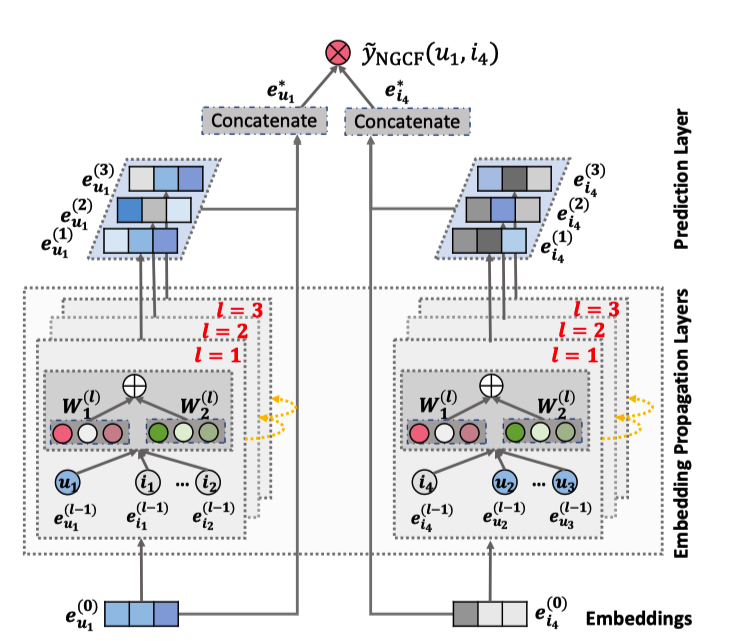user u 的递归公式如下：
$\mathbf{e}_{u}^{(l)}=\text { LeakyReLU }\left(\mathbf{m}_{u \leftarrow u}^{(l)}+\sum_{i \in \mathcal{N}_{u}} \mathbf{m}_{u \leftarrow i}^{(l)}\right) \\$

$\left\{\begin{array}{l} \mathbf{m}_{u \leftarrow i}^{(l)}=p_{u i}\left(\mathbf{W}_{1}^{(l)} \mathbf{e}_{i}^{(l-1)}+\mathbf{W}_{2}^{(l)}\left(\mathbf{e}_{i}^{(l-1)} \odot \mathbf{e}_{u}^{(l-1)}\right)\right) \\ \mathbf{m}_{u \leftarrow u}^{(l)}=\mathbf{W}_{1}^{(l)} \mathbf{e}_{u}^{(l-1)} \end{array}\right. \\$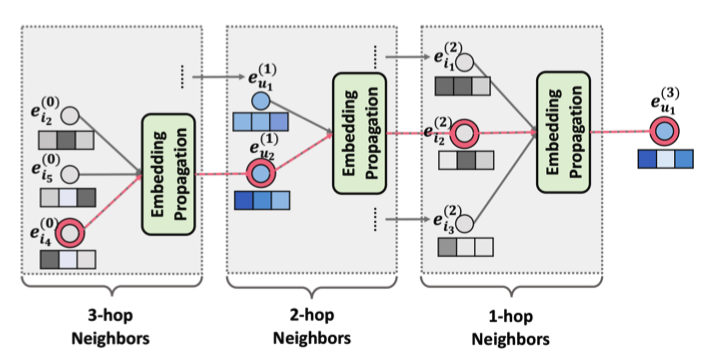$\mathbf{E}^{(l)}=\operatorname{LeakyReLU}\left((\mathcal{L}+\mathbf{I}) \mathbf{E}^{(l-1)} \mathbf{W}_{1}^{(l)}+\mathcal{L} \mathbf{E}^{(l-1)} \odot \mathbf{E}^{(l-1)} \mathbf{W}_{2}^{(l)}\right) \\$

$\mathcal{L}=\mathbf{D}^{-\frac{1}{2}} \mathbf{A} \mathbf{D}^{-\frac{1}{2}} \text { and } \mathbf{A}=\left[\begin{array}{cc} \mathbf{0} & \mathbf{R} \\ \mathbf{R}^{\top} & \mathbf{0} \end{array}\right] \\$

## 2.3 Prediction Layer

$\mathbf{e}_u^{*} = \mathbf{e}_u^{0} || \cdot \cdot \cdot ||\mathbf{e}_u^{L} \\$

$\hat{y}_{\text{NGCF}}(u,i) = {\mathbf{e}_u^{*}}^T \mathbf{e}_i^{*} \\$

## 2.4 Optimization

$Loss = − \sum_{(u,i,j) \in O}\ln \sigma(\hat{y}_{ui} − \hat{y}_{uj}) + \lambda||\Theta||_2^2 \\$

# 3.Experiment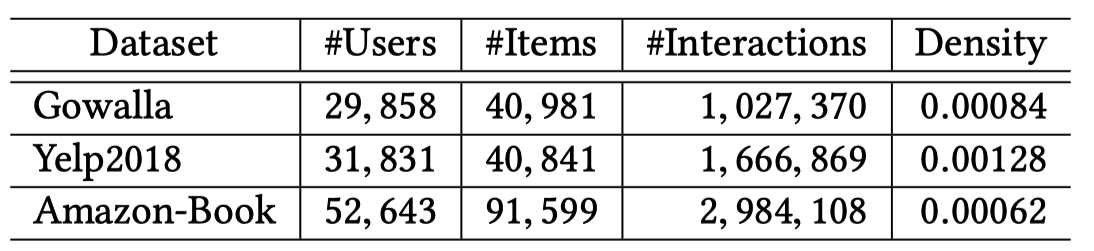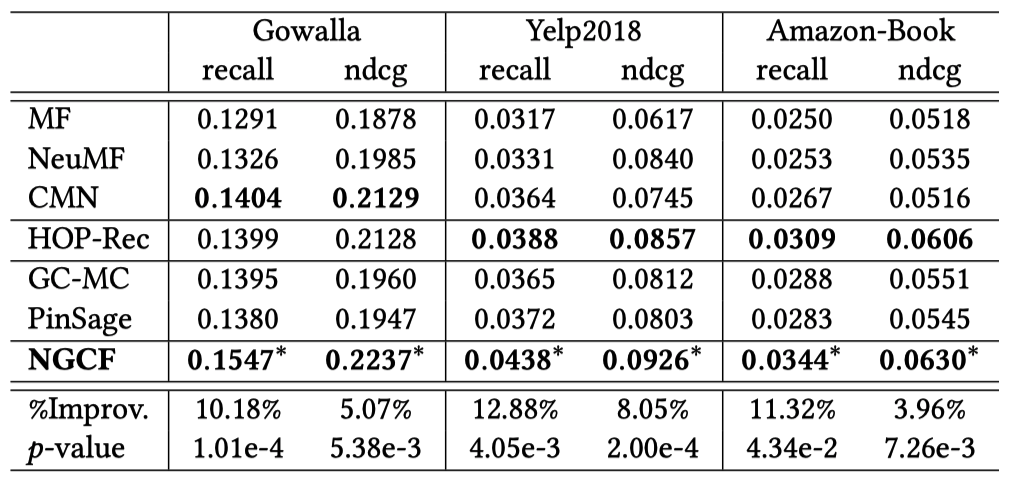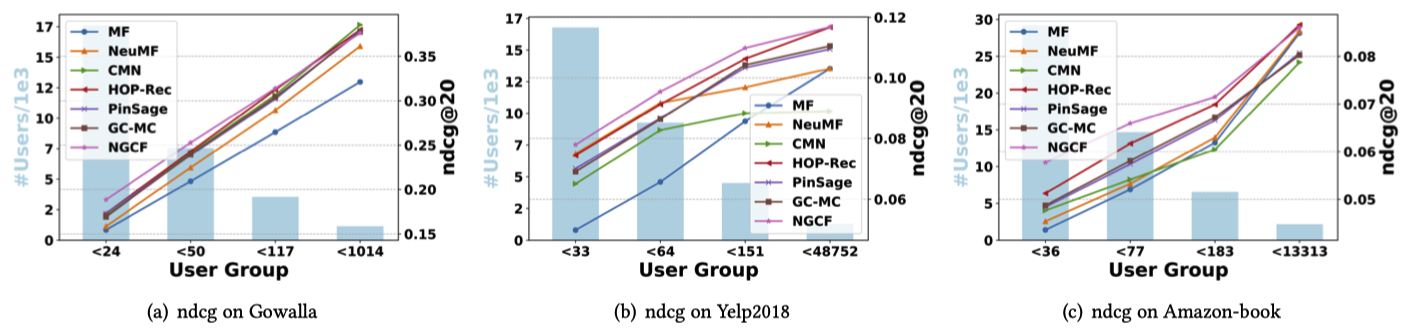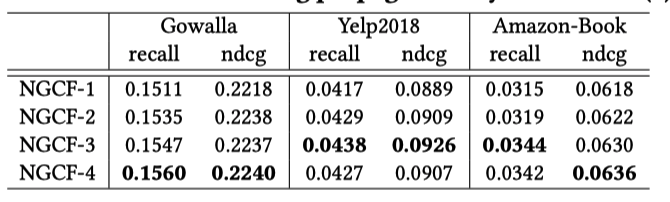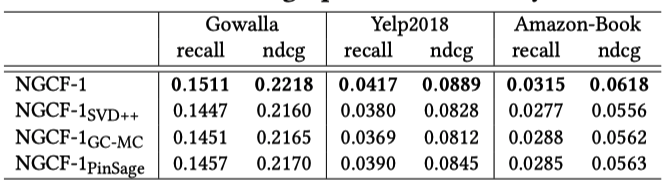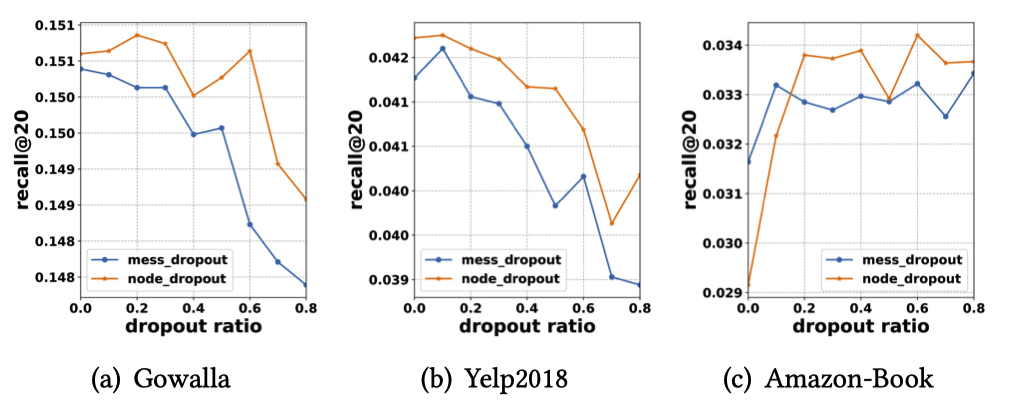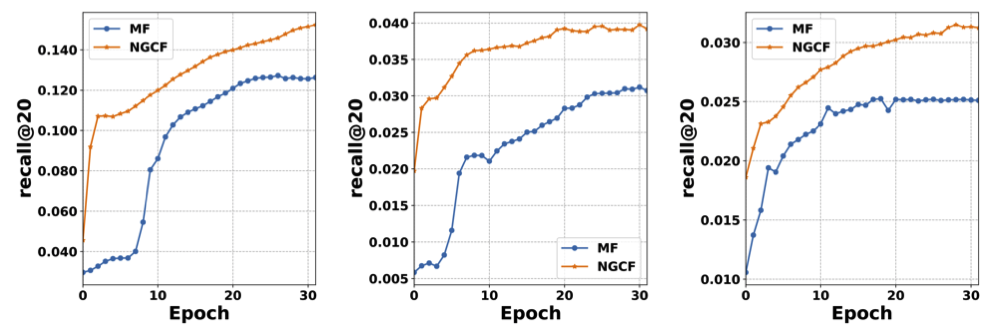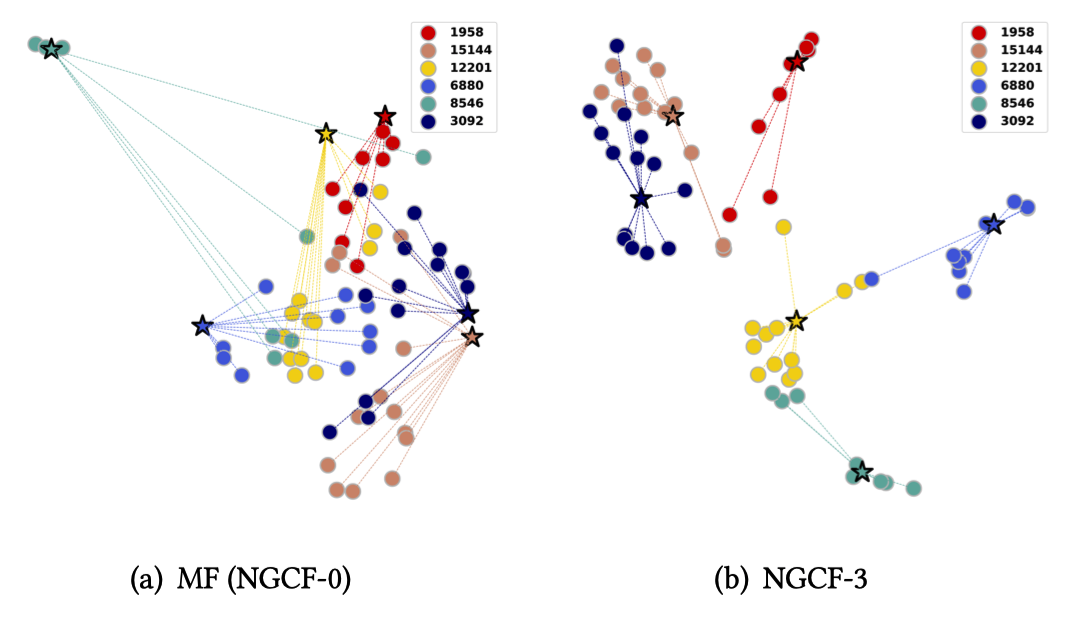# 5.Reference

1. Wang X, He X, Wang M, et al. Neural graph collaborative filtering[C]//Proceedings of the 42nd international ACM SIGIR conference on Research and development in Information Retrieval. 2019: 165-174.